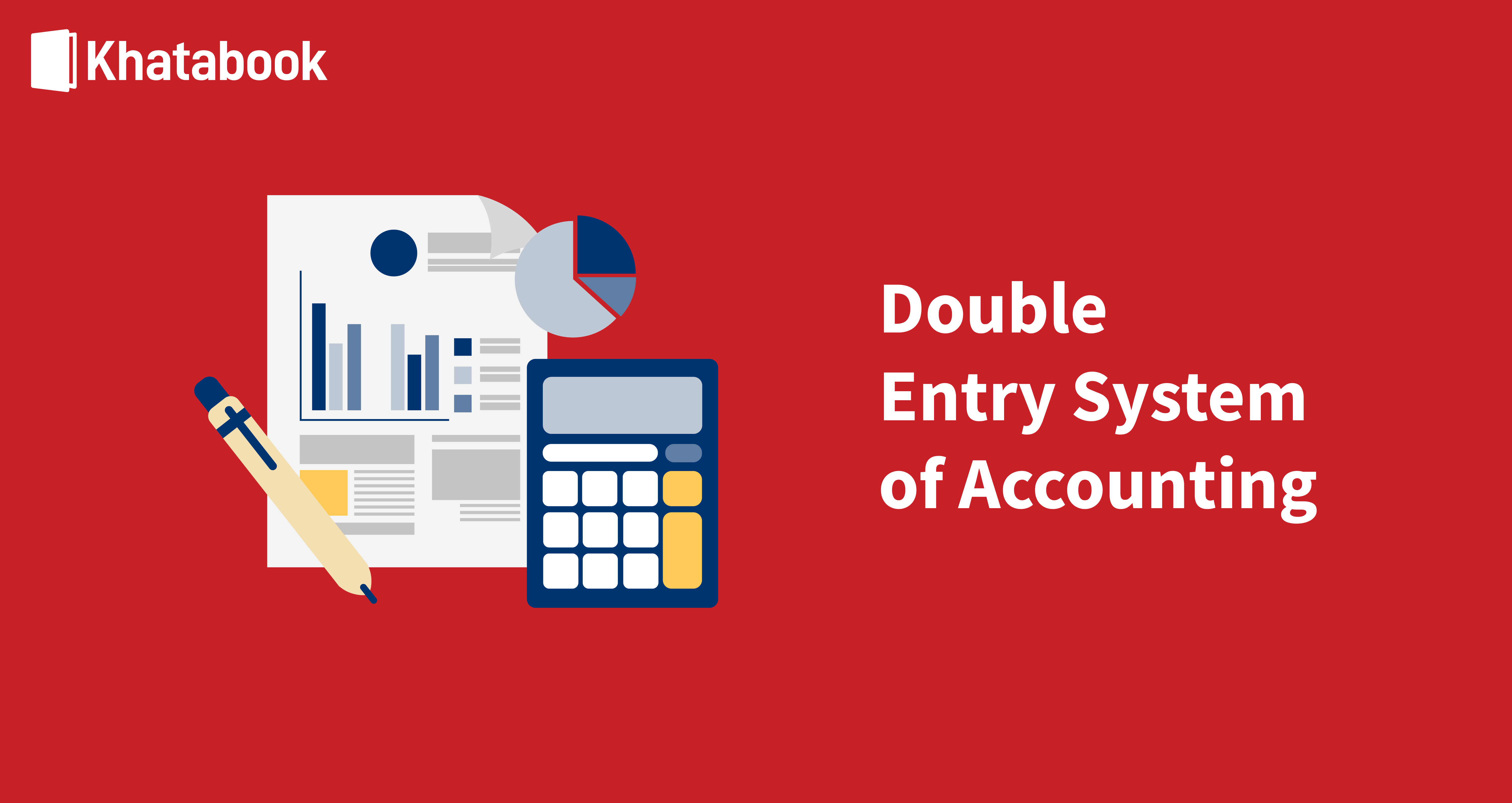# Know How To Calculate Cost of Capital With Examples

The primary objective of project evaluation in a business is achieved by determining the cost of capital. Various methods, terminology, and formulae are used in investment decisions, enabling a company to calculate the cost of capital, also known as the cut-off rate.

When a company’s projected Internal Rate of Return or IRR is high and it has exceeded the cost of capital, it means the potential for more profitability of an investment.  Therefore, it suggests that the net cash flows are higher. Investors look for higher IRR before investing in a company. Thus, the cost of capital refers to the foundation of evaluating the risks of a business's financial aspects defined in leverage ratio, which is discussed in this article. So, read on to know more!

## What is cost of capital?

What do you mean by cost of capital? The definition of cost of capital is the return rates of a company earned from its investments in the marketplace to increase its value. This is also the rate required by the capital's suppliers for their contribution to the company.

The four forms of cost of capital refer to in any company or business enterprise and are generally known as:

• Debts
• Equity Shares
• Preference Shares
• Retained Earnings.

Debts:

For the cost of capital, debt means the borrowed funds of a company that need to be repaid in the future or later. This form of capital growth denotes what the company raises as a loan, whether short-term or long-term, like overdrafts, machinery loans, etc.

Equity Shares:

Equity shares or equity cost of capital is invested by the investors and owners towards the company’s capital. The Equity capital is also known as ‘equity or 'share capital and is the total of the number of equity shares multiplied by its face value and forms the company’s equity share capital.

Preference Shares:

The issue of preference shares is a way of raising a company's cost of capital through the issue of preference shares. The primary difference between equity and preference shares is that the preference shares have a fixed dividend rate and have preferential rights over the profits and assets if the company faces liquidation.

Retained Earnings:

The cost of capital definition in retained earnings is the sum of profits earned by a business minus the value of stock dividends or other payable capital distributions. In financial statements, the account of retained earnings denotes the Owner or Member Capital found in the equity section of the balance sheet along with equity held by shareholders.

Does this mean that the owner's capital and retained earnings are the same? Note that the owner's equity and retained earnings denote the business ownership capital and can be different for different business forms like proprietorship, private limited, and others. The owner's equity is hence an account category, showing the business owner's company share. Retained earnings apply to all business forms, including corporations and firms.

## Financial Management and the Components of Cost of Capital:

The three crucial 'cost of capital financial management' factors connected to what the cost of capital means is explained below:

• Zero Risk-Return: This situation implies the expected rate of return of a business or company or its projects and involves no business or financial risks to the capital.
• Business Risk Premium: The concept of business risk is evaluated by capital budgeting decisions made by a company in its investment proposals. This implies that if a company selects a project having more than normal risks, the capital providers will expect or need their capital to have a higher rate of return exceeding the standard rates. Hence, premiums on business risks have an essential role in assessing capital costs and increases the Cost of Capital or its premiums in return rates. How the premiums are set depends on the selection of projects of a company, its objectives, goals, and how much the company wants to increase its market value.
• Financial Risk Premium: Since the company's pattern of capital structure involves financial risks, an assessment of the company's debt volumes is important. Companies with higher debt capital are considered riskier when compared to companies with lower debt capital.

### The formula for Cost of Capital:

The three costs of capital components mentioned above can also be written in equation form as follows:

If K is the Cost of Capital and r0 is the zero risk level, then r1 is the premium for business risk, and r2 is the premium for financial risk. The operating leverage ratio r1 measuring the business risk, and the financial leverage ratio r2 determine the combined leverage risk ratio measuring the total risk.

K the Ratio of Combined Leverage = r1 x r2 or (Ratio of Operating Leverage X Ratio of Financial Leverage).

### Types of company business risks:

• Economic Risk (These fluctuate with the markets and economy)
• Fraud and Security Risks
• Financial Risk
• Reputation Risk
• Comfort or Competition Risk
• Operational Risk

Companies can mitigate risks by using a proactive approach- identifying the risk hazards earlier on and taking risk mitigation steps before they occur. These measures can help avoid and spot risks detrimental to a business's health and raise the ratio of operating business leverage.

## Some ways of business risk mitigation are:

• Prioritise risk management and have a plan or policy to reduce threats, risks, thefts etc.
• Limit your liabilities and assess liability or risk reduction measures.
• Have a good Quality Assurance Program.
• Use a Controlled Growth Plan.
• Limit the number of High-Risk Customers.
• Have a Risk Appraisal and Management Team.

### Why the cost of capital is important:

The cost of capital is vital to potential buyers who use the weighted average cost of equity to help them assess acquiring a company or assigning a value to its cash flows in the near future, its risks, benefits, and expenses. The weighted average cost of capital or WACC formula results are coupled with indicators like the after-tax cost of debt and others to provide the company's best assessment under review.

The weighted average cost of equity or WACE also helps to assess the capital-intensive company campaigns and projects. This aids in determining how the overall earnings and their returns translate into shareholder earnings. This also provides a weighted index rather than the average overall figures or costs.

The WACE metric determines a particular equity type’s cost multiplied by its representative percentage of the total represented capital structure. Almost all formulae use the WACE for calculating the cost of equity. It is also a good measure for the company's new stock issuance when it decides to raise more capital in preferential shares, debentures etc. Bonds are also a cheap way of raising capital through debt. The WACC metrics provide the investors with the weighted and capital debt costs in an analysis of the company’s balance sheet.

## Calculating the WACE or Weighted Average Equity Cost:

Normally the WACE or weighted average cost of equity calculations involve more than the cost of debt calculations. Start with the cost of new commonly held stock, preferred stock cost and retained earnings costs separately. One of the best methods to do this involves the CAPM formula:

The cost of equity is given by the risk-free return rate  [beta x (rate of return market rate minus the risk minus the rate of return-free rate)].

Here, the beta risk rate is assumed from similarly performing companies. The equity cost of common stock retained earnings, and preferred stock is generally in a tight range. Let us use an example where the cost of common stock, retained earnings, and preferred stock are 14%, 11% and 12%, respectively. Now we need to calculate the portion of each form of equity in the total equity. Take an example of a company where the stock is apportioned as 50% for equity stock and 25% for preferred shares and retained earnings. Multiply the individual costs of each equity form of the total equity and then sum up these values; we arrive at 19.5% by the average pricing model. In this particular case,

WACE= (0.14 x 0.50) (0.11 x 0.25) (0.12 x 0.25) (0.12 x 0.25) = 0.1275 or approximately 12.8%.

Just averaging the equity costs across categories in the example above would give us an equity cost of 12.3%.

### Calculating the WACC Cost of Capital:

Generally, the weighted average cost is calculated which involves calculating the debt costs, the interest amount paid by a company, and its total debt.

Consider the following in a cost of capital formula example-

• Ko denotes the Capital Overall Cost
• Wd denotes the debt capital weight
• Wp indicates the preference share of capital weight
• Wr represents the retained earnings weight
• We denotes the equity share capital weight
• Kd denotes the debt specific cost
• Kp denotes the preference share capital specific cost
• Kr indicates the retained earnings specific cost
• Ke denotes the equity share capital specific cost

Calculating the cost of capital need not be confusing. Use the following methods and the example below to help understand the concept of cost of capital.

### A. Specific Capital Cost Computation:

Thus to get the Specific Cost of the capital sources, one has to sum up the four costs associated with the capital sources. Namely,

• Debt cost
• Preference shares cost
• Equity shares cost
• Retained earnings cost

The specific cost of capital formula cost Ks is given by (Kd Kp Kr Ke).

### B. WACC method of computation of the Capital Composite Cost:

In this method, the Composite Capital is used. The Average Composite Capital or the different sources of capital combined cost, when taken together, is arrived at using the weighted method, also called the WACC or the Weighted Average Cost of Capital. The formula used in the calculation of WACC is as below and best explained with an example.

## WACC cost of capital formula:

The Overall Cost of Capital, Ko, is given by the sum of the individual costs multiplied by their weights.

Ko = [(Kd into Wd) (Kp into Wp) (Kr into Wr) (Ke into We)].

Overall Capital Cost, Ko = Sum of [(Kd the debt specific cost multiplied by Wd the debt capital weight) (Kp denotes the preference share capital specific cost multiplied by Wp the preference share of capital weight) (Kr the retained earnings specific cost multiplied by Wr the retained earnings weight) (Ke the equity share capital specific cost multiplied by We the equity share capital weight)].

Don’t get confused. Let’s look at an example.

### WACC method example:

Calculate the Cost of Capital for Company A from the data of cost capital structure employed by it in its project financing.

 Amount (Rs) Cost After-tax  (%) Weights Capital from Equity shares 8,50,000 16% 0.3269 Capital from Retained earnings 4,50,000 15% 0.173 Capital from Preference shares 6,50,000 12% 0.25 Capital from Debentures 6,50,000 9% 0.25 Total Capital 26,00,000

Calculation of WACC or Cost of capital of Company A:

 Source Amount (Rs) denoted by (1) Weights (Specific Capital/Total cost) denoted by (2) After-tax Cost (Cost%/100) denoted by (3) Weighted Cost denoted by (4) = (2) into (3) Equity share capital 8,50,000 0.3269 0.16 0.0523 Retained earnings 4,50,000 0.173 0.15 0.02595 Preference share capital 6,50,000 0.25 0.12 0.03 Debentures 6,50,000 0.25 0.09 0.0225 Total 26,00,000 0.13075

The WACC or Weight of Average Cost of Capital here is 13%. This means that Company A employs a weighted average capital cost of 13%. This also means that the company pays its capital lenders a 13% premium as a risk return.

You can check out the formula from the tabulated results, which will be the same.

WACC = [( 8,50,000 / 24,00,000) * 0.16 ( 4,50,000 / 26,00,000 ) * 0.15 (6,50,000 / 26,00,000) * 0.12 (6,50,000 / 26,00,000) * 0.09] = 13%.

Thus, if the projected rate of return is higher than the WACC, this means the company investors make a justifiable rate of return on their investments.

Also Read: Double Entry System of Accounting

## Conclusion:

This article shows how the cost of capital is important to investors and the health indices of a company trying to raise capital. By using the formula, you can efficiently calculate the cost of capital for an organisation. You can make this process easier by using Biz Analyst. This application helps in significant business growth for Tally users where adequate analysis of sales and growth can be determined along with doing efficient data entry among others functions.

## FAQs

1. Is the cost of capital and rate of return the same?

The cost of capital is the company’s expected returns on its shares and securities. However, the IRR or Investor rate of return is the premium on the investment that makes the investor risk justifiable.

2. How is the WACC useful?

The WACC or weighted average cost of capital is the figure that provides the rate of return that shareholders or lenders may expect to receive for providing the company with its capital. It determines whether the company is losing or building value in the market. In general, the rate of investment returns on invested capital must be higher than the company value of its WACC to make the investment profitable to investors.

3. Why is the cost of capital important?

Calculating the cost of capital is an accounting and economical tool to arrive at the costs of an investment opportunity. It should generally maximise the rate of returns to potential investors. The cost of capital also defines the cash flows into the specific investment or business project.

4. What are the main costs of capital types evaluated?

The five types of Cost of Capital generally calculated can be said to be

• Capital Implicit Cost
• Capital Explicit Cost
• Capital Specific Cost
• WACC or Weighted Average Cost of Capital
• WACE Capital Marginal Cost

5. What is the difference between WACC and Cost of Capital

The cost of capital is derived as the total capital costs of equity and debt. In contrast, the weighted average of costs or WACC is evaluated as a weighted proportion of equity and debt held in a business.

6. Name an application that can help in the calculation of the Cost of Capital

Biz Analyst is one such application that can aid in the process of accounting such as calculation of Cost of Capital. You can also stay connected with your business, reduce payment delays and increase sales team productivity.

## Related Posts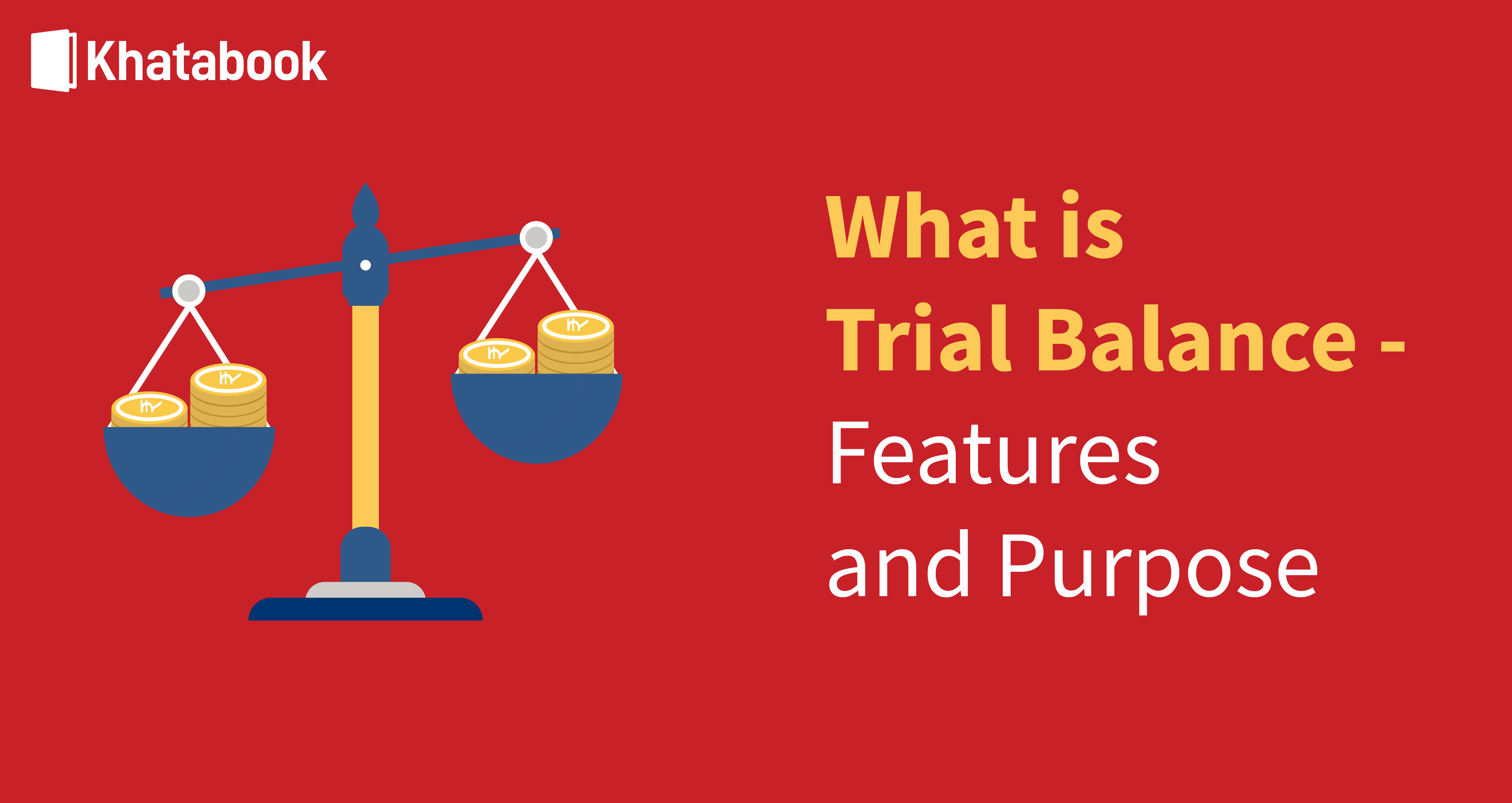### What is Trial Balance - Meaning, Features and Purpose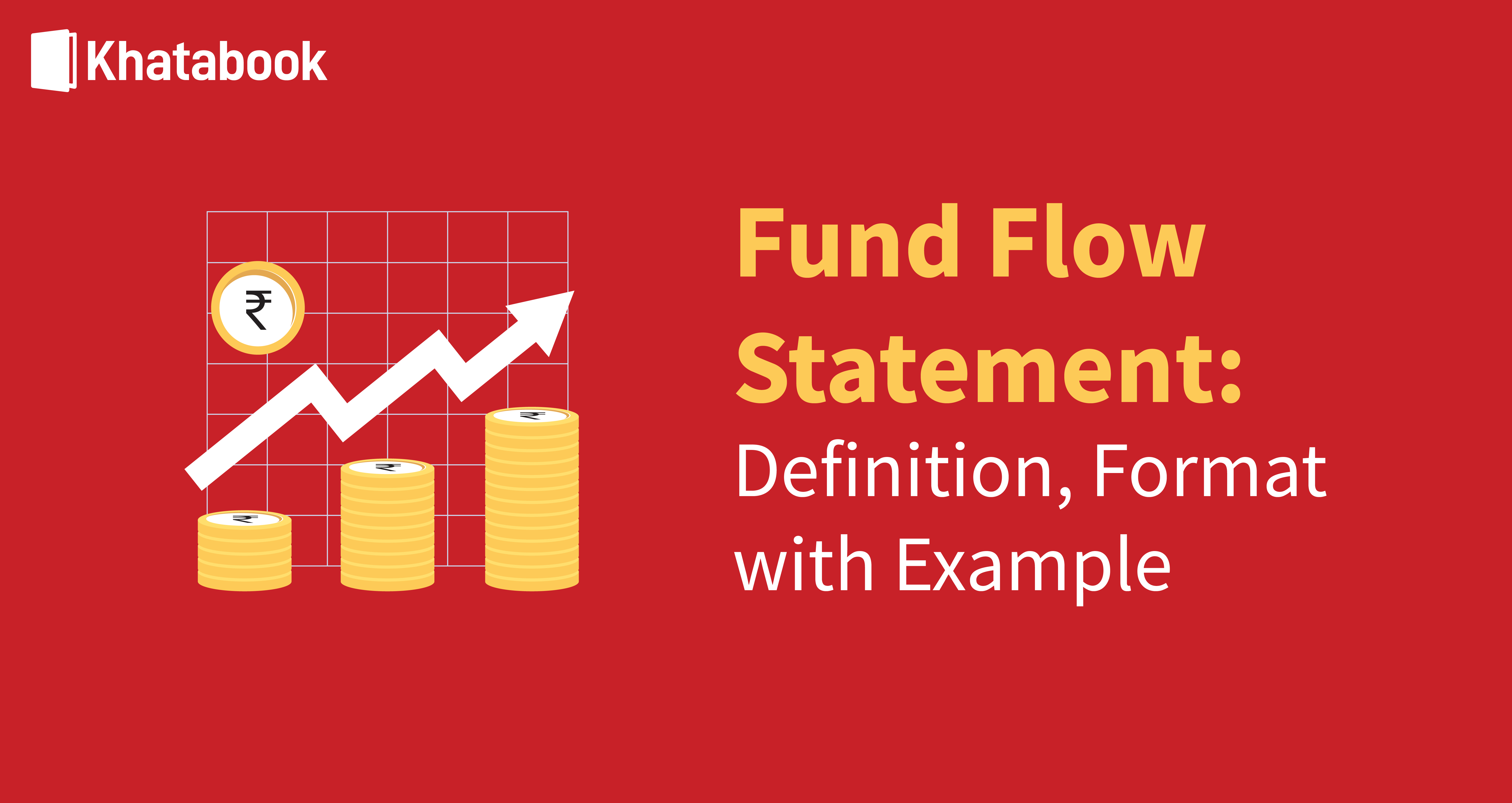### Fund Flow Statement - Meaning, Format And Examples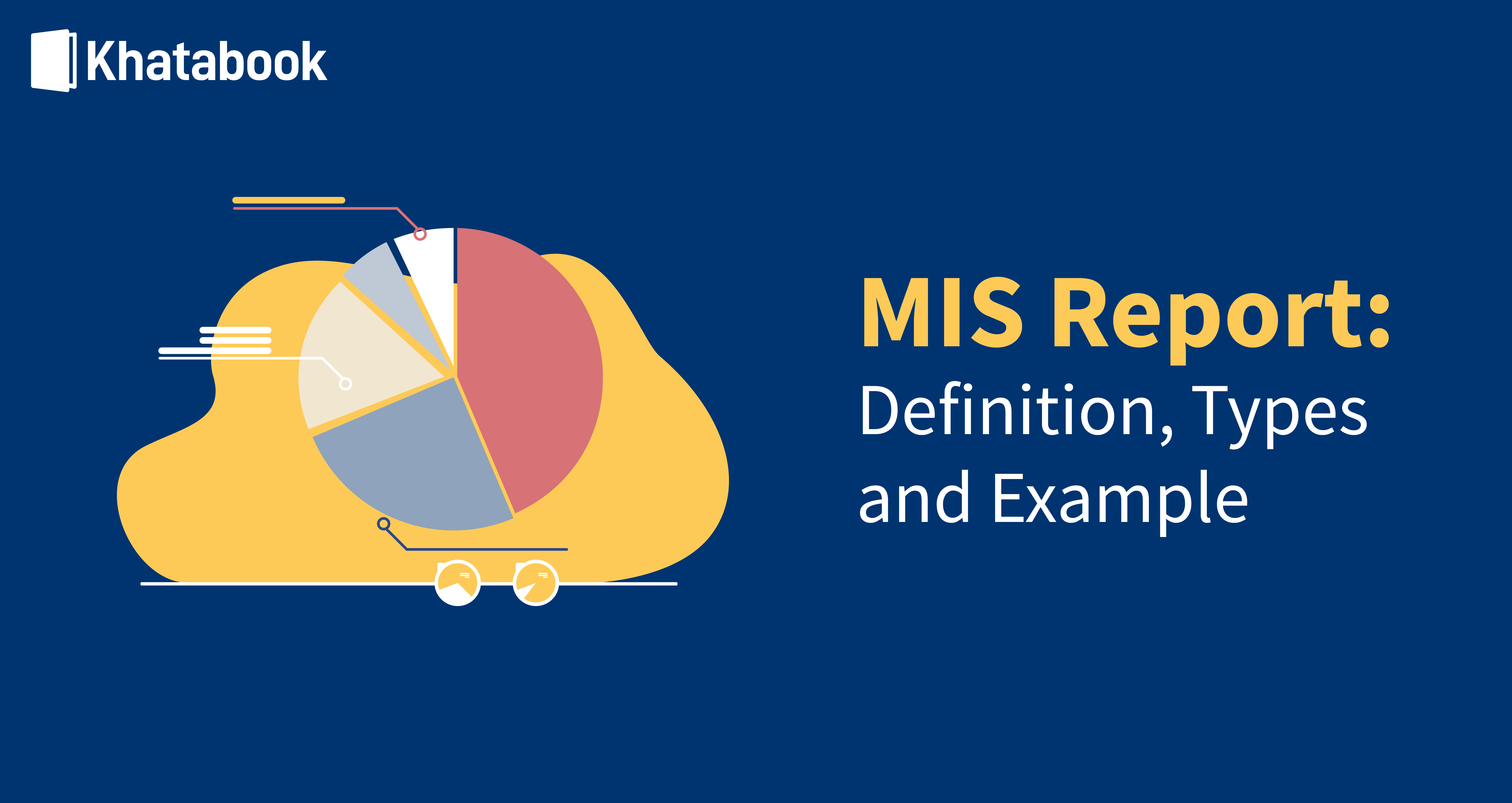### Everything You Need To Know About MIS Report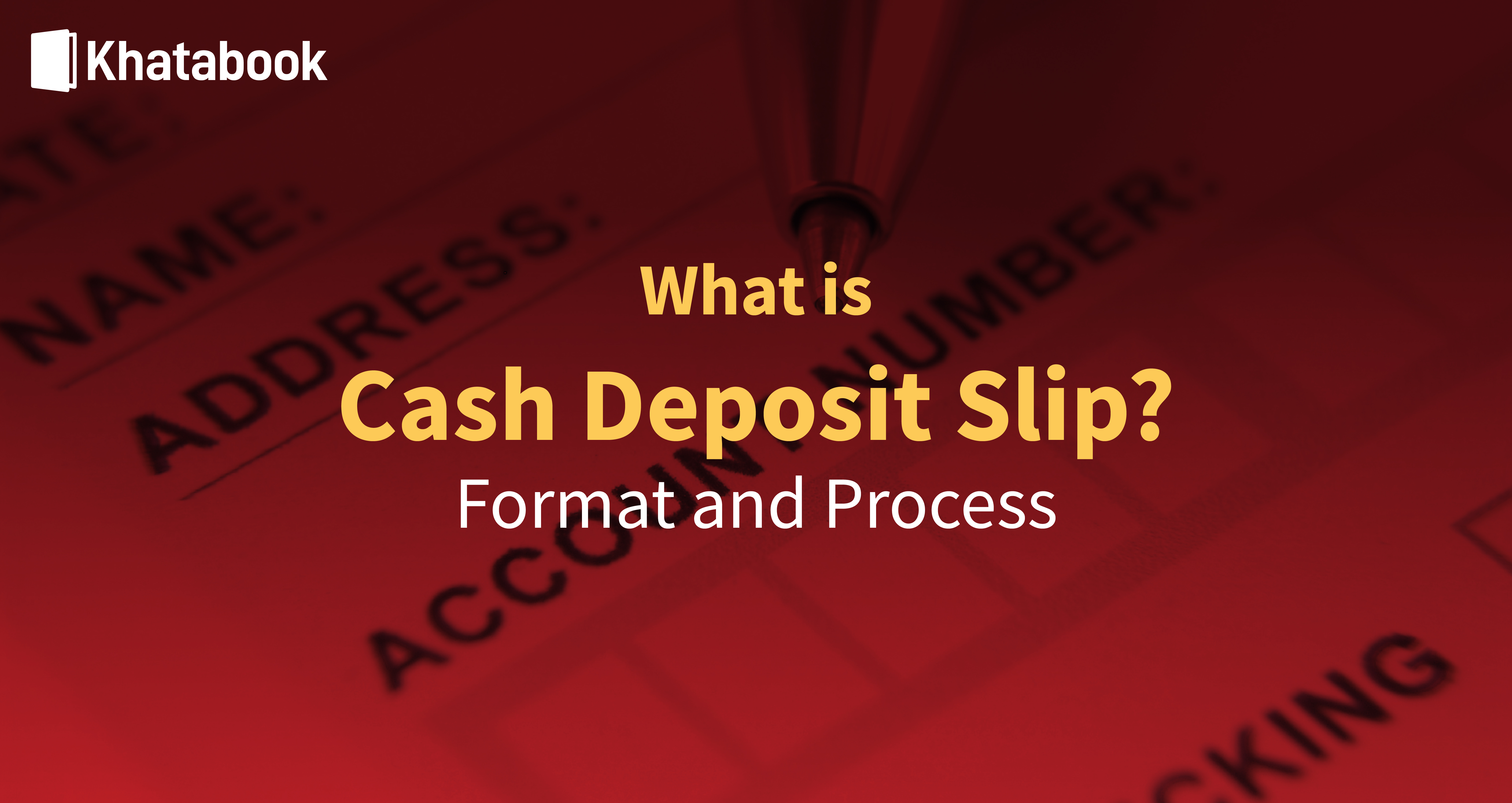### All You Need To Know About Cash Deposit Slip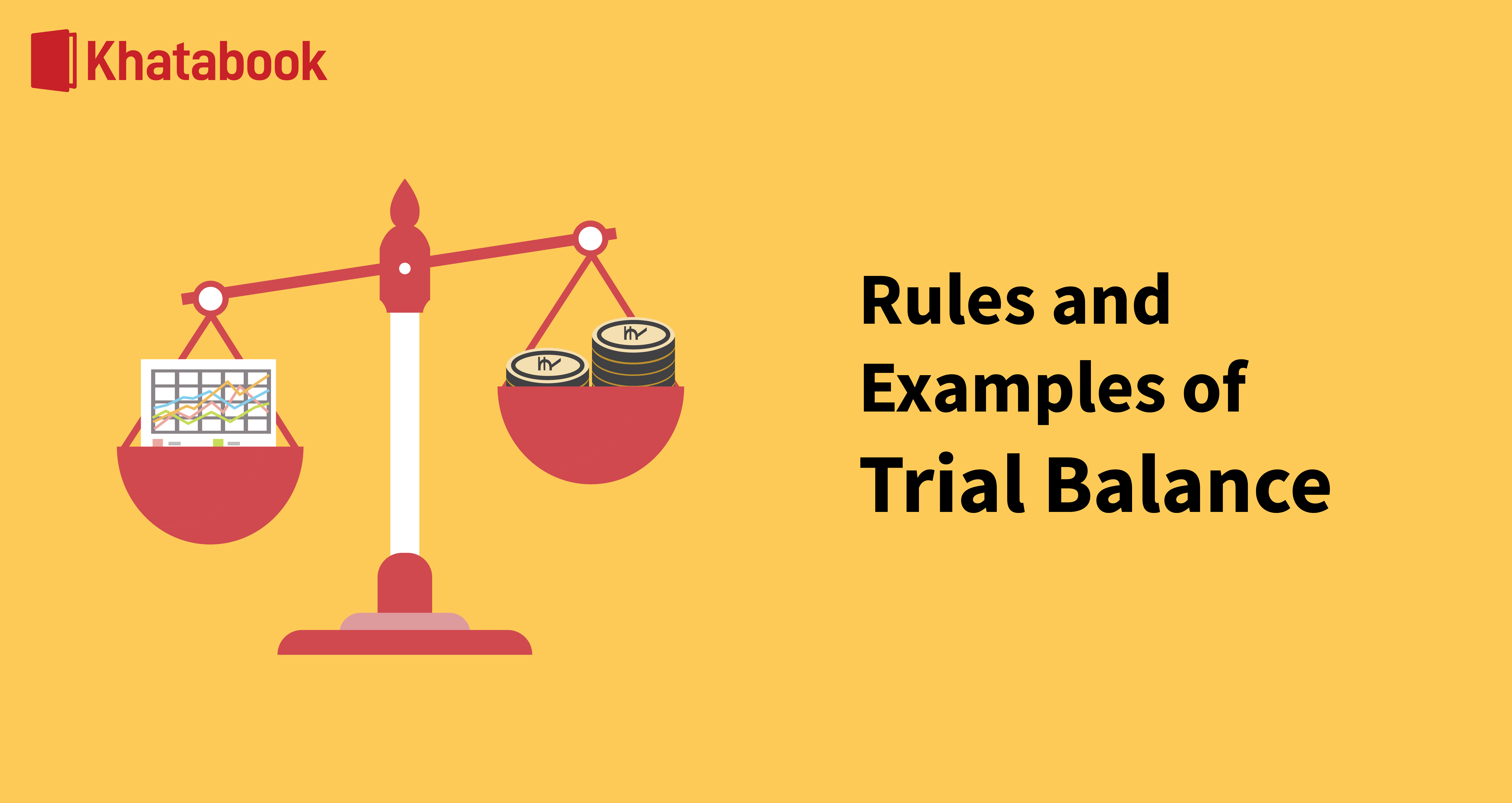### Trial Balance: Rules Explained With Examples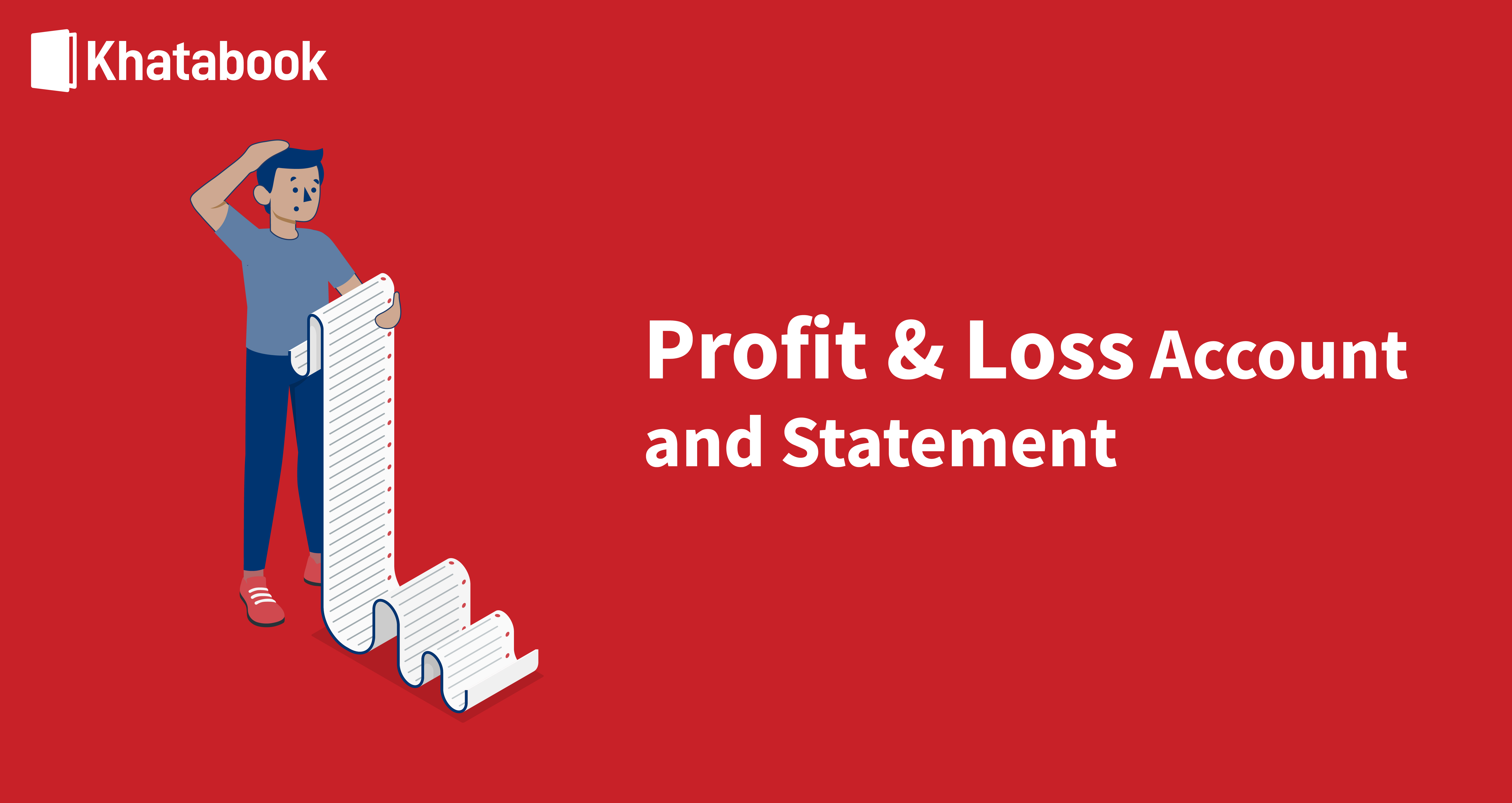### Profit and Loss Account & Statement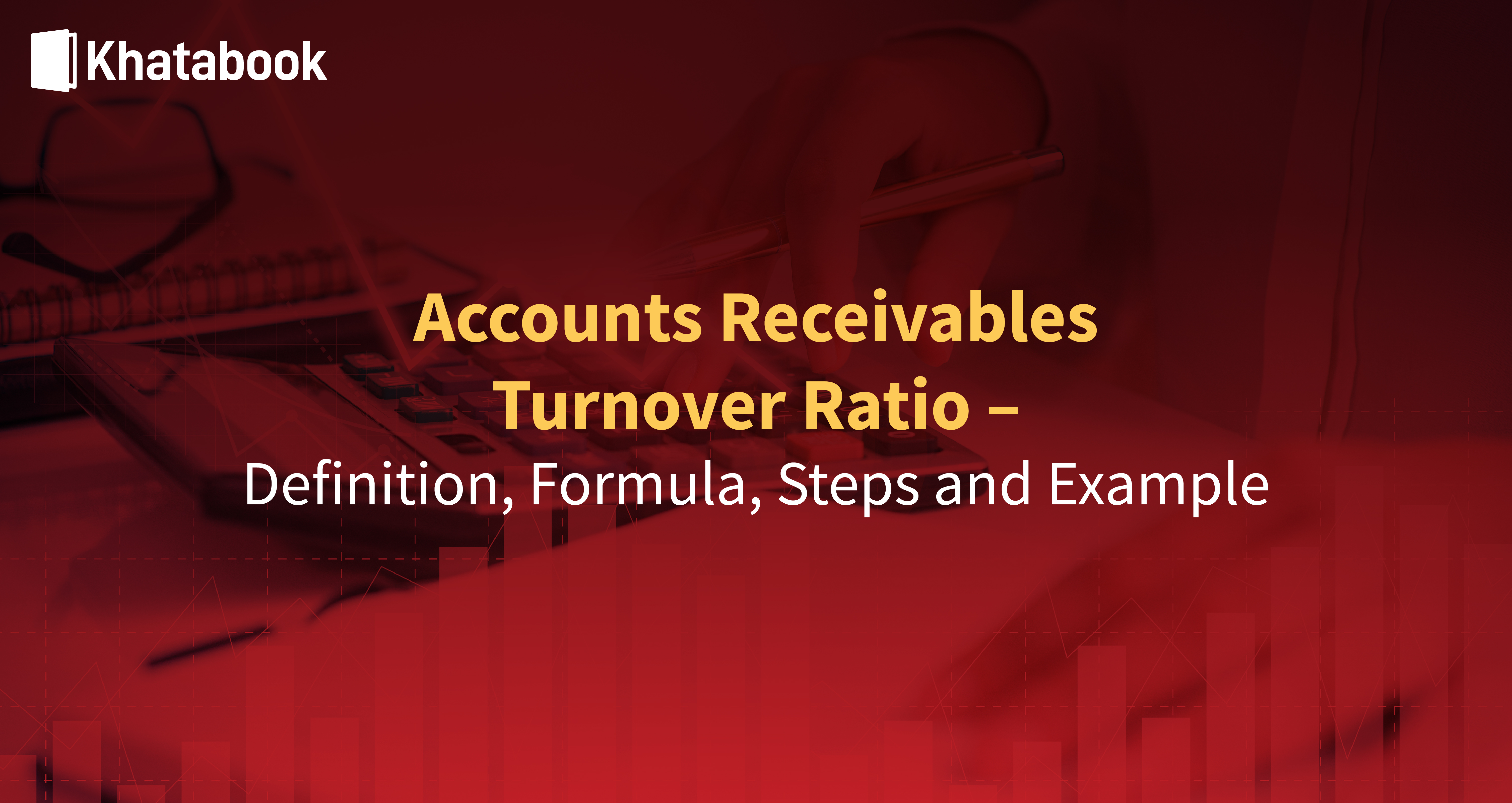### Step-By-Step Guide To Calculate Accounts Receivable Turnover Ratio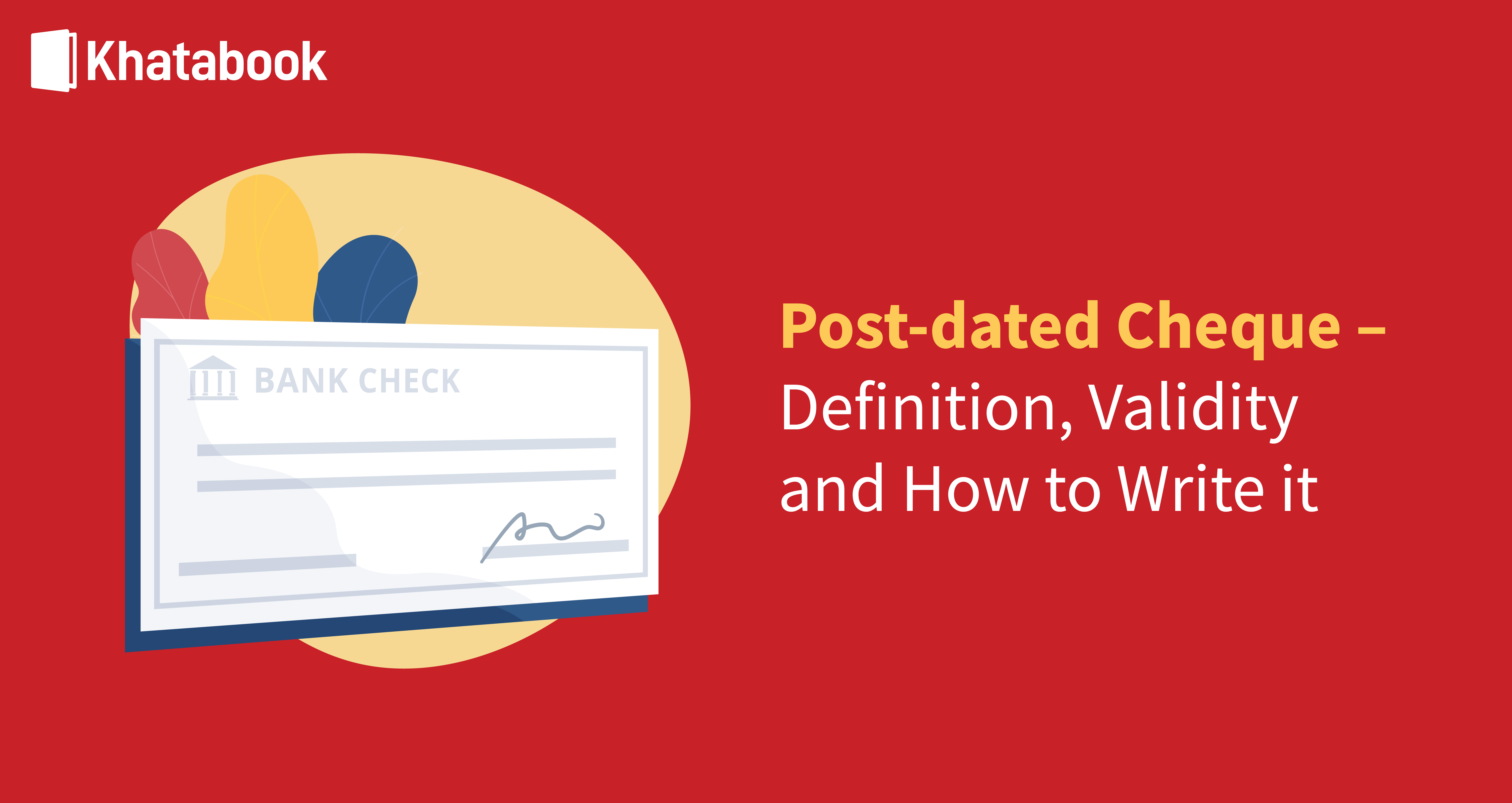### All You Need To Know About Post-dated Cheques